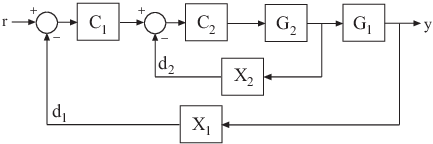## Extract Responses from Tuned MATLAB Model at the Command Line

This example shows how to analyze responses of a tuned control system by using `getIOTransfer` to compute responses between various inputs and outputs of a closed-loop model of the system. You can obtain other responses using similar functions such as `getLoopTransfer` and `getSensitivity`.

Consider the following control system.Suppose you have used `systune` to tune a `genss` model of this control system. The result is a `genss` model, `T`, which contains tunable blocks representing the controller elements `C1` and `C2`. The tuned model also contains `AnalysisPoint` blocks that represent the analysis-point locations, `X1` and `X2`.

Analyze the tuned system performance by examining various system responses extracted from `T`. For example, examine the response at the output, y, to a disturbance injected at the point d1.

```H1 = getIOTransfer(T,'X1','y'); ```

`H1` represents the closed-loop response of the control system to a disturbance injected at the implicit input associated with the `AnalysisPoint` block `X1`, which is the location of d1:`H1` is a `genss` model that includes the tunable blocks of `T`. `H1` allows you to validate the disturbance response of your tuned system. For example, you can use analysis commands such as `bodeplot` or `stepplot` to analyze `H1`. You can also use `getValue` to obtain the current value of `H1`, in which all the tunable blocks are evaluated to their current numeric values.

Similarly, examine the response at the output to a disturbance injected at the point d2.

```H2 = getIOTransfer(T,'X2','y'); ```

You can also generate a two-input, one-output model representing the response of the control system to simultaneous disturbances at both d1 and d2. To do so, provide `getIOTransfer` with a cell array that specifies the multiple input locations.

```H = getIOTransfer(T,{'X1','X2'},'y'); ```